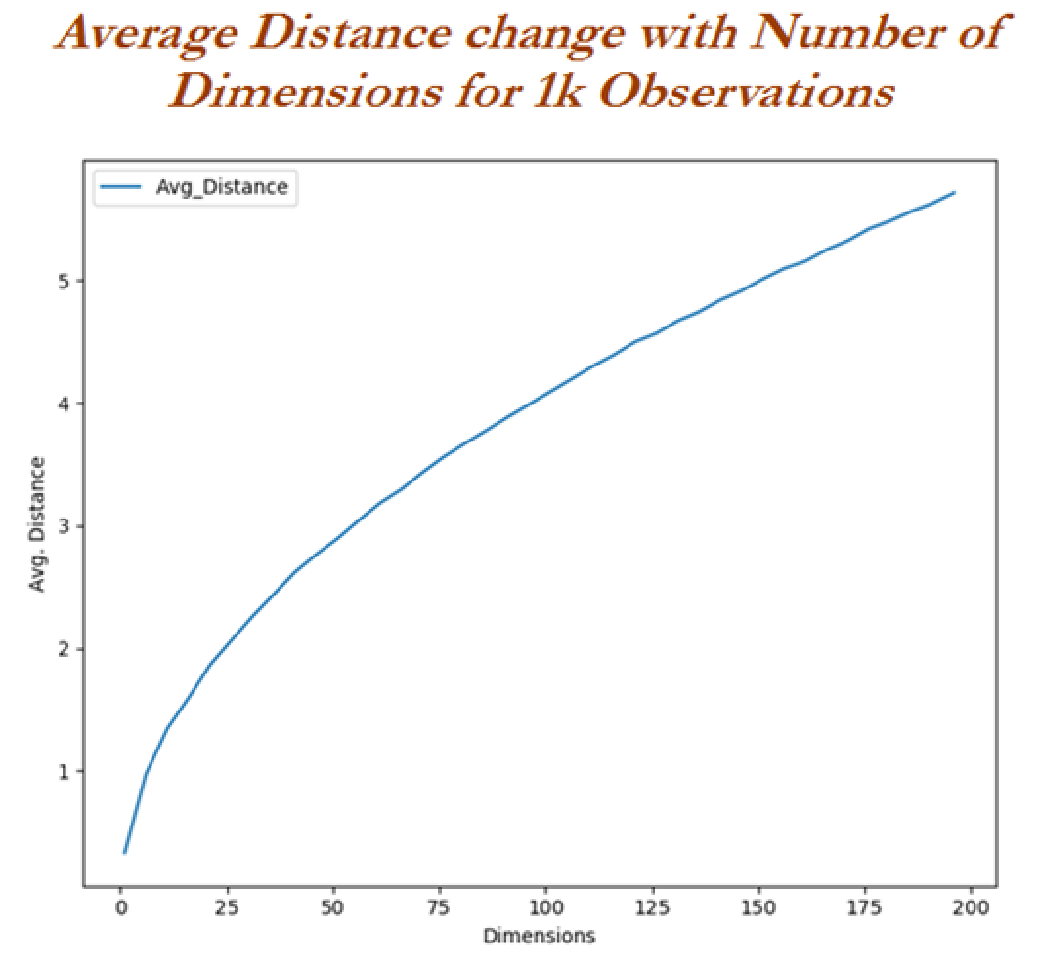# Explain Curse of dimensionality

697    Asked by SumikoLacoste in Data Science , Asked on Dec 17, 2019

Curse of dimensional mostly occurs in algorithms like KNN which completely depends on distances. High dimensional spaces are obviously largely and points in such spaces disperse from each other. We will evaluate the curse of dimensional with 1000 observations with the change in dimensions.

Let us import the libraries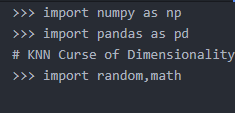Let us generate random numbers between 0 and 1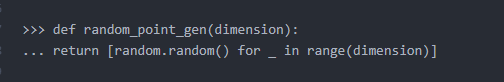Now we will calculate root mean sum of squares of Euclidean distances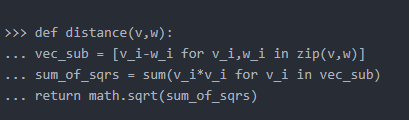Let us calculate the distance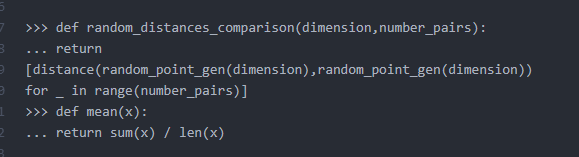Now we plot the observations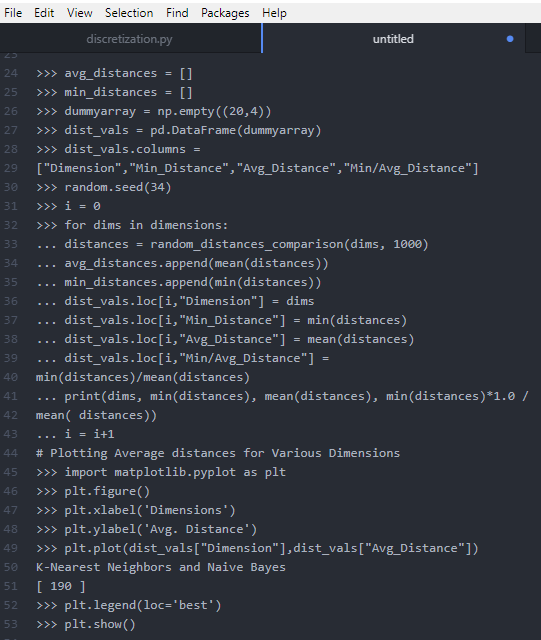The output of the observation is given below